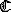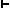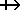## BASES FOR KEY SYSTEMS AND STRUCTURES STUDIED IN THIS BOOK

(A) Non-Modal Propositional Calculi

(A.1) Standard ('Hilbert-style') axiomatizations
Rules of inference: All systems in this section (A.1) will have detachment and substitution for variables as postulated rules of inference.

(A.1.1) Intuitionistic propositional calculus (IC): axioms Cl and C2 are a basis for positive (intuitionistic) implication (ICI)

 C1: CCpCqrCCpqCpr C2: CpCqp K1: CpCqKpq K2: CKpqp K3: CKpqq A1: CpApq A2: CqApq A3: CCprCCqrCApqr N1: CCpNpNp N2: CNpCpq
Alternatively, take constant false proposition Æ rather than N as primitive, and define Na as Cao, replacing axioms Nl and N2 by:

 Æ1: CÆp
(A.1.2) Classical propositional calculus (PC). For C, K, A, and N (or Æ) primitive, add the following to IC:

 C3: CCCpqpp
Cl (or syl, CCpqCCqrCpr), C2, and C3 give full classical implication. For C and N primitive, use

 CN1: CCpqCCqrCpr CN2: CpCNpq CN3: CCNppp
For K and N primitive, use:

 KN1: CpKpp KN2: CKpqp KN3: CCpqCNKqrNKrp
Definitions of other connectives in the latter two systems are as usual.
(A.2) Non-modal sequent-logic
The distinction between intuitionistic (LIC) and classical (LPC) sequent-logic is made by the provisos on certain of the rules below.

Structural rules:

rule affecting antecedent affecting succedent
weakening
 G ® Q a, G ® Q
 G ® Q G ® Q, a
with Q empty intuitionistically
contraction
 a, a, G ® Q a, G ® Q
 G ® Q a, a G ® Q, a

 G ® Q, a a, D ® L

CUT
G, D ® Q, L
Logical rules:

Rule introduces: Into Antecedent Into Succedent
C
 G ® Q°, a b, G ® Q

Cab, G  ® Q
 a, G ® Q, b G ® Q, Cab

with Q° = Q for the Classical System; empty otherwise

K
 a, b, G ® Q Kab, G ® Q
 G ® Q, a G ® Q, b

® Q, Kab
A
 a, G ® Q° b, G ® Q

Aab, G  ® Q
 G ® Q, a°, b° G ® Q, Aab

with one of a°, empty intuitionistically; both present classically

N
 G ® Q, a Na, G ® Q
 a G ® Q G ® Q, Na

with Q empty intuitionistically

(A.3) Semantic tableaux for PC (structure MPC)

C-l:
 When Cab occurs on the left of a tableau, split the tableau, writing a on the right of one of the resulting tableaux, and b on the left of the other For intuitionistic tableaux only, the tableau receiving a on its right is to omit any formula on the original's right side upon which action is pending.
C-r: When Cab occurs on the right of a tableau, write a on the left and b on the right of that tableau.
Κ-l: When Kab occurs on the left of a tableau, write both a and b on the left of that tableau.
K-r: When Kab occurs on the right of a tableau, split that tableau (alternatively), writing a on the right of one of the tableaux thus formed and b on the right of the other.
A-l: When Aab occurs on the left of a tableau, split that tableau, writing a on the left of one of the tableaux thus formed, and b on the left of the other.
Α-r:
 If Aab occurs on the right of a classical tableau, write both a and b on the right of that tableau. If Aab occurs on the right of an intuitionistic tableau, split that tableau conjunctively, and write a on the right of one of tableaux resulting from the split, and b on the right of the other.
N-l:
 If Na occurs on the left of a classical tableau, write a on the right of that tableau. If Na occurs on the left of an intuitionistic tableau, write a on the right of that tableau, provided there is no formula already on the right with action pending on it.
Ν-r: If Na occurs on the right of a tableau, write a on the left of that tableau.

(B) Modal Calculi

(B.1) 'Lewis-style' axiomatizations of S1°-S5: S1°-S1; S2°-T; S3°-S3; S4°-S5
These axiomatizations have K, N, and M primitive (L defined as NMN andab as NMKaNb). Rules of inference are substitution for variables, strict detachment, adjunction, and substitutivity of strict equivalence.
Axioms for S1°:

 M1:KpqKqp M2:Kpqp M3:KKpqrKpKqr M4:pKpp M5:Kpqqrpr
Axioms for S2°:
M1-M5 plus

 M7:MKpqMp
Axioms for S3°:
M1-M5 plus

 M8:pqMpMq
Axioms for S4°:
M1-M5 plus

 M9:MMpMp
Axioms for :
Where a is any PC thesis, add LLa to S1°
Axioms for Sl, S2, S3, S4, T:

 M6:pMp
to the respective 'nought system'.
Axioms for S5:
M1-M5 plus

 M10:MpLMp

(B.2) 'Lemmon-style' bases for S1°-S5; S1°-T; S3°-S5
These axiomatizations have C, N, and L primitive (M defined as NLN andas LC). They are all based on classical PC; axioms for PC may be taken as CN1, CN2, and CN3, with substitution for variables and material detachment. In addition to PC, the bases are as follows:
Basis for Sl°:
Axiom:

 L1: CKLCpqLCqrLCpr
Rules of Inference:

RL1:
 Ifa thenLa, provided either (a) a is a PC thesis, or (b) a is of form CKLbLgd, whereLAbg.
BKW:   If bothab andba, thenLaLb.
RLE:   IfLa, thena.

Basis for S2°:
Axiom:

 L2: CLCpqCLpLq
Rules of Inference:

 RLE: IfLa, thena (same as Sl°) RL2: If a is a PC theorem or a theorem of form CLbg, thenLa
Basis for :
Same as for S2°, except replace RL2 by:

 RL: Ifa, thenLa
Basis for S3°:
Axiom:

 L4: CLCpCqrLCLpCLqLr
Rules of Inference:
RLE and RL2 as for S2°

Basis for S4°:
Same as for S3°, except replace RL2 with RL as in .

Bases for S1, S2, S3, S4:
Replace rule RLE in the respective 'nought' system with axiom

 L3: CLpp

 . . ., or (c) a is of form CLbb
For S3 and S4, axiom L4 may be replaced by axiom

 L5: CLCpqLCLpLq

Basis for S5:
Add to T (or to any of the systems of this section (B.2)) axiom

 L6: CMLpLp

(B.3) Systems between S4 and S5, and Sobocinski's 'non-Lewis modal' systems
These systems may be considered to be based on the S4 of the above section. They are as follows:

 S4.1 = S4 + M13,pLppCMLpp or M14.pLpLpCMLpLp S4.1.4 = S4 + M15,pLppCLMLpp S4.2 = S4 + M12,MLpLMp S4.2.1 = S4.2 + M13 S4.3 = S4 + M11, ALpqLqp D (S4.3.1) = S4.3 + M13 S4.3.2 = S4 + M17, ALpqCMLqp S4.3.3 = D + M20, CLMpCLMqCMKpqLMKpq S4.3.4 = S4.3.2 + M20 S4.4 = S4 + M16,pCMLpLp S4.9 = S4.4 + M20 = S4 + M21,CpLMpCCqLMqCMKpgLMKpq = S4.4 + M18, ACMLppCLMqMLq = S4 + M19, AMLpLpMLMqCqLq V1 = S4 + M24, ALpApqpNq V2 = S5 + M24 K1 = S4 + M22,LMpMLp K2 = S4.2 + M22 K3 = S4.3 + M22 K3.1 = D + M22 K3.2 = S4.3.2 + M22 K4 = S4.4 + M22
(B.4) Modal sequent-logic for S1°-S5
The modal sequent-logics are based on the logic LPC of section A.2. The sequent-logics other than LS1°, LS2°, LT°, LS3°, and LS4° (sequent-logics corresponding to the 'nought systems') will have a rule to introduce L into the antecedent:

 a, G ® Q L® La, G ® Q
The sequent-logics corresponding to the nought systems will lack this rule, having only a rule to insert L into the succedent. Other than this distinction between nought and nonnought systems, modal sequent-logics will be distinguished by their ®L rule, as follows:

LS1 and LS1°:

 D ® Q G ® a

LG ® La

 1 If G is singular or empty, Q = G and D = a; 2 In all other cases, D is empty and Q has as its members two and only two of the formulas belonging to G.

LS2 and LS2°:

 G ® a ®L LG ® La
®L may be applied with the sequence G empty; however, if it is once so applied, it may not be applied again at all in the same proof string.

LT and LT°:
Rule ®L for these systems is as for LS2 and LS2°, but the restriction is omitted.

LS3 and LS3°:

 D, G ® LQ, a D, LG ® a

®L
LD, LG ® La

 (a) Each formula of LQ is a subformula of a or a subformula of LG or LD; the left premiss is provable in LPC. (b) Either D or G may be empty, but if both of these sequences are empty, rule ®L may not be applied again in the same proof string.

LS4 and LS4°:

 D, LG ® a ®L LD, LG ® La
No restrictions on G, D.

LS5:

 D, LG ® LQ, a ®L LD, LG ® LQ, La
Normal-form theorem not provable.

(B.5) Sequent-logic for systems between S4 and S5
Sequent-logics have been developed for several of these systems. The normal-form theorem is in general not provable for these systems, so CUT must be included in their formulations.
LS4.2:
Replace LS4's ®L by:

 D, LG ® LNLQ, a ®L LD, LG ® LNLQ, La

LS4.3:
Add the following sequent as an axiom schema to LS4:

LALab, LAaLb ® La, Lb

LS4.4:
Axioms and rules involving the arrow ® are as for LS4; in addition, another arrowis employed; axioms and rules forare obtained by replacing ® in axioms and rules of LS5 by. Sequents GQ occurring in proofs are called 'subtheorems'; only sequents involving the ordinary arrow are theorems. Movement from subtheorems to theorems is by the rule

 LGLQ CONV Q, LGLQ

(B.6) Tableaux for modal systems
All these structures include MPC. Tableaux for the nought systems (i.e. tableaux of the structures MS1°, MS2°, MS3°, MS4°, and MT°) differ from those for other systems in that the auxiliarity relation is not reflexive; tableaux are not auxiliary to themselves. In the other structures discussed, this relation is reflexive; this affects the application of the rules for L on the left. We shall state rules asserting what to do when a formula La occurs left or occurs right in a tableau. Distinguishing the system MSn° from MSn depends on the difference noted in their auxiliarity relation.

MT° and MT:
For MT°, the only tableaux which must be auxiliary to a given tableau are those constructed by an application of L-r in that tableau; for MT in addition, each tableau is auxiliary to itself:

 L-r: If La occurs on the right of a tableau, construct a new tableau auxiliary to that tableau and beginning with only a on its right. L-l: If La occurs on the left of a tableau, write a on the left of each tableau auxiliary to that tableau.

MS2° and MS2:
Structures for S2 and S2° will involve possible worlds of a kind different from the ordinary, called 'non-normal' worlds, and so also non-normal tableaux. Statements La are always false in a non-normal world, and statements Ma are always true. These structures are like MT° and MT except for having the following instead of L-r above:

 L-r(2): When La occurs on the right of a (normal) tableau, split the alternative set to which that tableau belongs; in one of the new alternative sets begin a new auxiliary normal tableau with a on its right; in the other new alternative set, begin a new non-normal tableau with a on its right.

MS1° and MS1
These are of considerable complication, and the reader is referred to the relevant place in Chapter 9.

MS4° and MS4:
Here the accessibility and so the auxiliarity relation is transitive; to reflect this, we alter rule L-l of MT° and MT to read

 L-l(4): If La occurs on the left of a tableau t (of LS4° or LS4), write both a and La on the left of each tableau auxiliary to t (unless, of course, a formula to be written is already there).

MS3° and MS3:
Replace rule L-l for MS2° and MS2 with:

 L-l(3): When La occurs on the left of a (normal) tableau t, write both a and La on the left of each normal tableau auxiliary to t (unless, of course, the formula to be written already stands there). Write only a on the left of each non-normal tableau auxiliary to t.

MS5:
In MS5 the accessibility and auxiliarity relations are reflexive, transitive, and symmetrical. The rules employed are:

 L-r(5): If the formulas La, LQ are all the formulas beginning with L on the right of a tableau, create an auxiliary tableau beginning with a, LQ on the right. L-l(5): If La occurs left in a tableau t, write a and La left in every tableau ancestrally generated from t (unless such formulas already occur there).

MS4.2:
The accessibility relation here is 'convergent' as well as transitive and reflexive. Replace L-r of MS4 by:

 L-r(4.2¢): When the formulas LNLQ, La are all the formulas beginning with L on the right of an MS4.2¢ tableau, construct a tableau auxiliary to that tableau and having LNLQ and  a on its right.

MS4.3
The accessibility relation here is reflexive, transitive, and linear. Replace L-r in MS4 by

 L-r(4.3): Where La1, . . ., Lan  occur on the right of an MS4.3 tableau, split that tableau into n tableaux, each one having auxiliary to it a tableau with a different one of the a1, . . ., an on its right; the auxiliary tableau with ai on its right, 1 £ i £ n, will also have the set of formulas La1, . . ., Lan less Lai on its right.

MS4.4:
Here the main and the auxiliary tableaux differ in kind: a rule for L-left is given for main and for auxiliary tableaux respectively:

 L-l(4.4m): If La occurs left in a main MS4.4 tableau, write La and a left in all tableaux of that alternative set (except where they already occur). L-l(4.4a): If La occurs left in an auxiliary MS4.4 tableau, write La and a left in all auxiliary tableaux of that alternative set (except where they already occur).
And in like manner, rules for L on the right, here for auxiliary and for main tableaux respectively:

L-r(4.4a): If La occurs left in an auxiliary tableau in MS4.4, begin a new auxiliary tableau with a on its right.
L-r(4.4m):
 (1) If La occurs right in a main tableau and a also occurs right there, no further action is taken with this La. (2) If La1, . . ., Lam. occur right in a main tableau and a1, . . ., am all occur left in that tableau, begin a new tableau auxiliary to the main and starting with La1, . . ., Lam on its right. (3) If La1, . . ., Lan occur right in a main tableau and none of the a1, . . ., an. occur either left or right in that tableau, split that tableau into n + 1 alternative tableaux; a different one of the a1, . . ., an will be written on the right of each of n of these tableaux; all the a1, . . ., an will be written on the left of the (n + 1)th.